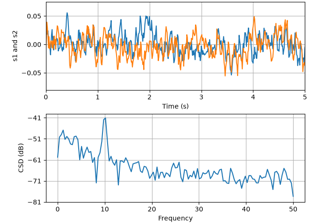# matplotlib.axes.Axes.csd#

Axes.csd(x, y, NFFT=None, Fs=None, Fc=None, detrend=None, window=None, noverlap=None, pad_to=None, sides=None, scale_by_freq=None, return_line=None, *, data=None, **kwargs)[source]#

Plot the cross-spectral density.

The cross spectral density $$P_{xy}$$ by Welch's average periodogram method. The vectors x and y are divided into NFFT length segments. Each segment is detrended by function detrend and windowed by function window. noverlap gives the length of the overlap between segments. The product of the direct FFTs of x and y are averaged over each segment to compute $$P_{xy}$$, with a scaling to correct for power loss due to windowing.

If len(x) < NFFT or len(y) < NFFT, they will be zero padded to NFFT.

Parameters:
x, y1-D arrays or sequences

Arrays or sequences containing the data.

Fsfloat, default: 2

The sampling frequency (samples per time unit). It is used to calculate the Fourier frequencies, freqs, in cycles per time unit.

windowcallable or ndarray, default: window_hanning

A function or a vector of length NFFT. To create window vectors see window_hanning, window_none, numpy.blackman, numpy.hamming, numpy.bartlett, scipy.signal, scipy.signal.get_window, etc. If a function is passed as the argument, it must take a data segment as an argument and return the windowed version of the segment.

sides{'default', 'onesided', 'twosided'}, optional

Which sides of the spectrum to return. 'default' is one-sided for real data and two-sided for complex data. 'onesided' forces the return of a one-sided spectrum, while 'twosided' forces two-sided.

The number of points to which the data segment is padded when performing the FFT. This can be different from NFFT, which specifies the number of data points used. While not increasing the actual resolution of the spectrum (the minimum distance between resolvable peaks), this can give more points in the plot, allowing for more detail. This corresponds to the n parameter in the call to fft. The default is None, which sets pad_to equal to NFFT

NFFTint, default: 256

The number of data points used in each block for the FFT. A power 2 is most efficient. This should NOT be used to get zero padding, or the scaling of the result will be incorrect; use pad_to for this instead.

detrend{'none', 'mean', 'linear'} or callable, default: 'none'

The function applied to each segment before fft-ing, designed to remove the mean or linear trend. Unlike in MATLAB, where the detrend parameter is a vector, in Matplotlib it is a function. The mlab module defines detrend_none, detrend_mean, and detrend_linear, but you can use a custom function as well. You can also use a string to choose one of the functions: 'none' calls detrend_none. 'mean' calls detrend_mean. 'linear' calls detrend_linear.

scale_by_freqbool, default: True

Whether the resulting density values should be scaled by the scaling frequency, which gives density in units of 1/Hz. This allows for integration over the returned frequency values. The default is True for MATLAB compatibility.

noverlapint, default: 0 (no overlap)

The number of points of overlap between segments.

Fcint, default: 0

The center frequency of x, which offsets the x extents of the plot to reflect the frequency range used when a signal is acquired and then filtered and downsampled to baseband.

return_linebool, default: False

Whether to include the line object plotted in the returned values.

Returns:
Pxy1-D array

The values for the cross spectrum $$P_{xy}$$ before scaling (complex valued).

freqs1-D array

The frequencies corresponding to the elements in Pxy.

lineLine2D

The line created by this function. Only returned if return_line is True.

Other Parameters:
dataindexable object, optional

If given, the following parameters also accept a string s, which is interpreted as data[s] (unless this raises an exception):

x, y

**kwargs

Keyword arguments control the Line2D properties:

Property

Description

agg_filter

a filter function, which takes a (m, n, 3) float array and a dpi value, and returns a (m, n, 3) array and two offsets from the bottom left corner of the image

alpha

scalar or None

animated

bool

bool

clip_box

Bbox

clip_on

bool

clip_path

Patch or (Path, Transform) or None

color or c

color

dash_capstyle

CapStyle or {'butt', 'projecting', 'round'}

dash_joinstyle

JoinStyle or {'miter', 'round', 'bevel'}

dashes

sequence of floats (on/off ink in points) or (None, None)

data

(2, N) array or two 1D arrays

drawstyle or ds

{'default', 'steps', 'steps-pre', 'steps-mid', 'steps-post'}, default: 'default'

figure

Figure

fillstyle

{'full', 'left', 'right', 'bottom', 'top', 'none'}

gapcolor

color or None

gid

str

in_layout

bool

label

object

linestyle or ls

{'-', '--', '-.', ':', '', (offset, on-off-seq), ...}

linewidth or lw

float

marker

marker style string, Path or MarkerStyle

color

float

color

markerfacecoloralt or mfcalt

color

markersize or ms

float

markevery

None or int or (int, int) or slice or list[int] or float or (float, float) or list[bool]

mouseover

bool

path_effects

AbstractPathEffect

picker

float or callable[[Artist, Event], tuple[bool, dict]]

pickradius

unknown

rasterized

bool

sketch_params

(scale: float, length: float, randomness: float)

snap

bool or None

solid_capstyle

CapStyle or {'butt', 'projecting', 'round'}

solid_joinstyle

JoinStyle or {'miter', 'round', 'bevel'}

transform

unknown

url

str

visible

bool

xdata

1D array

ydata

1D array

zorder

float

psd

is equivalent to setting y = x.

Notes

For plotting, the power is plotted as $$10 \log_{10}(P_{xy})$$ for decibels, though $$P_{xy}$$ itself is returned.

References

Bendat & Piersol -- Random Data: Analysis and Measurement Procedures, John Wiley & Sons (1986)

## Examples using matplotlib.axes.Axes.csd#CSD Demo

CSD Demo